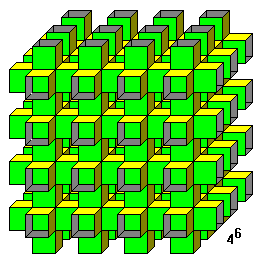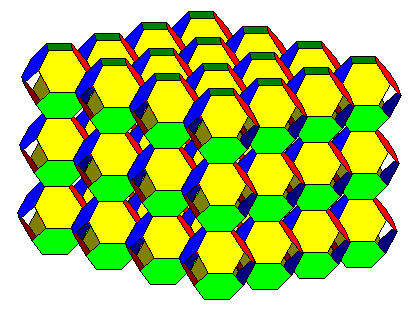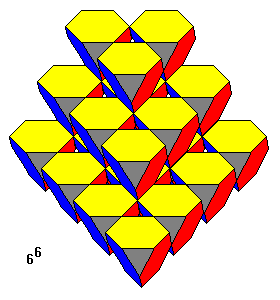# "Hyperbolic" Tesselations

Steven Dutch, Professor Emeritus, Natural and Applied Sciences, University of Wisconsin - Green Bay

If polygons are joined so that their angles at a vertex sum to less than 360 degrees, they form a polyhedron. If their angles sum to exactly 360 degrees they form a plane tesselation. But what happens if their angles sum to more than 360 degrees? Obviously there have to be at least four polygons, otherwise one or more of them would have to bend to fit. If we fit, say, four regular hexagons around a vertex, they form a saddle shape, that is, a negatively-curved surface.

Such tesselations have been explored a number of times and variously termed "pseudopolyhedra" and "infinite skew polyhedra". Somebody not already familiar with the literature on polyhedra might have a hard time figuring out what the terms mean or finding information on them. Thus, I call them "hyperbolic" tesselations, since they are at least approximations to tesselations on a hyperbolic plane.

## Coxeter Skew polyhedra

Geometer H. S. M. Coxeter referred to the three tesselations here as "infinite skew polyhedra" and considered them to be regular in the same sense as the Platonic solids and regular plane tesselations. They have regular faces and identical, symmetrical vertices.One of the simplest tesselations of this sort is a network of cubes with six squares meeting at each vertexTruncated octahedra can be joined to make a tesselation with four hexagons meeting at a vertex.Truncated tetrahedra with the trianglar faces omitted can be joined to form a tesselation with 6 hexagons meeting at each vertex.

## Gott's "Pseudopolyhedra"

J.R. Gott rediscovered the three tilings above in 1967 and also discovered a few others, which he termed "pseudopolyhedra." His remaining tilings do not qualify as infinite tilings by some criteria because they all include adjacent coplanar polygons.The above example consists of two parallel planes with a triangular tiling, joined by octahedral tunnels. It really isn't completely three-dimensional, though it is uniform in the sense that every vertex is the same.We can modify the previous structure to a true three-dimensional tiling by adding another set of octahedral tunnels to the other side of each plane and repeating the pattern.

## More "Hyperbolic" Tesselations

"Hyperbolic" Tesselations of A.F. Wells